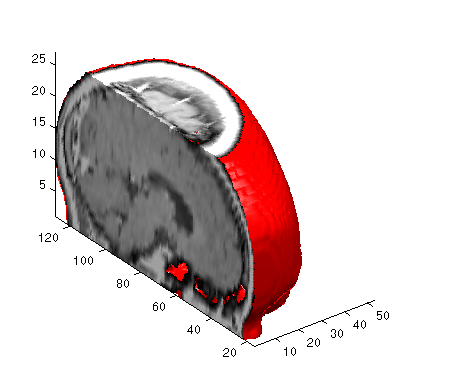MATLAB Function Referenceisocaps

Compute isosurface end cap geometry

Syntax

• ```fvc = isocaps(X,Y,Z,V,isovalue)
fvc = isocaps(V,isovalue)
fvc = isocaps(...,'`enclose`')
fvc = isocaps(...,'`whichplane`')
[f,v,c] = isocaps(...)
isocaps(...)
```

Description

```fvc = isocaps(X,Y,Z,V,isovalue) ``` computes isosurface end cap geometry for the volume data `V` at isosurface value `isovalue`. The arrays `X`, `Y`, and `Z` define the coordinates for the volume `V`.

The struct `fvc` contains the face, vertex, and color data for the end caps and can be passed directly to the `patch` command.

```fvc = isocaps(V,isovalue) ``` assumes the arrays `X`, `Y`, and `Z` are defined as `[X,Y,Z] = meshgrid(1:n,1:m,1:p)` where `[m,n,p] = size(V)`.

```fvc = isocaps(...,'enclose') ``` specifies whether the end caps enclose data values above or below the value specified in `isovalue`. The string `enclose` can be either `above` (default) or `below`.

```fvc = isocaps(...,'whichplane') ``` specifies on which planes to draw the end caps. Possible values for `whichplane` are `all` (default), `xmin`, `xmax`, `ymin`, `ymax`, `zmin`, or `zmax`.

```[f,v,c] = isocaps(...) ``` returns the face, vertex, and color data for the end caps in three arrays instead of the struct `fvc`.

```isocaps(...) ``` without output arguments draws a patch with the computed faces, vertices, and colors.

Examples

This example uses a data set that is a collection of MRI slices of a human skull. It illustrates the use of `isocaps` to draw the end caps on this cutaway volume.

The red `isosurface` shows the outline of the volume (skull) and the end caps show what is inside of the volume.

The `patch` created from the end cap data (`p2`) uses interpolated face coloring, which means the gray `colormap` and the light sources determine how it is colored. The isosurface patch (`p1`) used a flat red face color, which is affected by the lights, but does not use the colormap.

• ```load mri
D = `squeeze`(D);
D(:,1:60,:) = [];
p1 = patch(isosurface(D, 5),'FaceColor','red',...
'EdgeColor','none');
p2 = patch(isocaps(D, 5),'FaceColor','interp',...
'EdgeColor','none');
view(3); axis tight; daspect([1,1,.4])
colormap(gray(100))
camlight left; camlight; lighting gouraud
isonormals(D,p1)```

See Also

`isosurface`, `isonormals`, `smooth3`, `subvolume`, `reducevolume`, `reducepatch`

Isocaps Add Context to Visualizations for more illustrations of isocaps

Volume Visualization for related functions

© 1994-2005 The MathWorks, Inc.